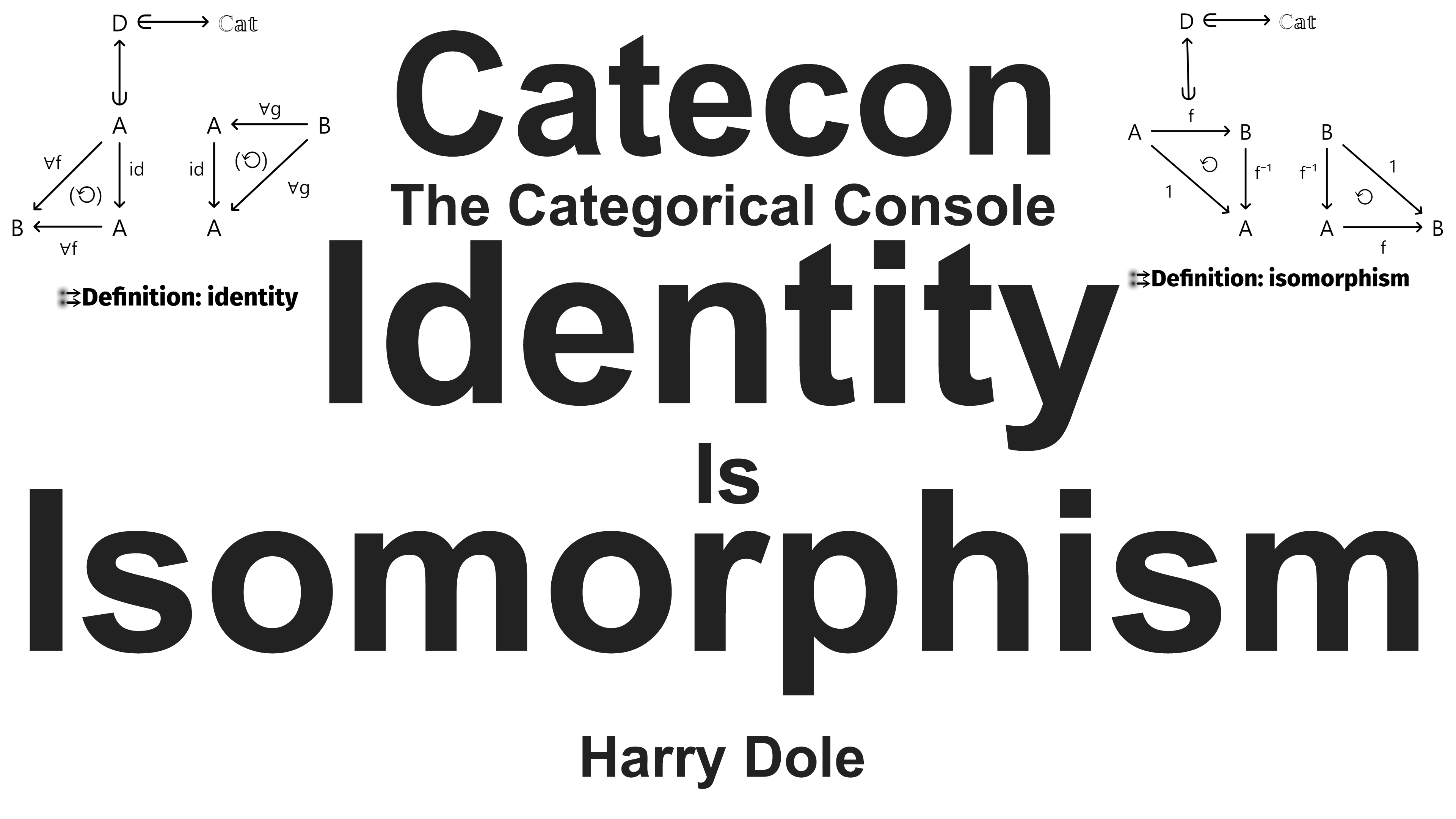# Catecon: Identity Is Isomorphism

See diagrams at https://catecon.net/d/hdole/identity and https://catecon.net/d/hdole/isomorphism.

Today, let’s define the notions of identity and isomorphism.

Then show in Catecon how an identity is an isomorphism.

Let’s get morphing!

Create a new diagram called identity.

CT’s say “The morphism id on an object A in the category D is an identity if for every f with domain A that f composed with id is f, and for every g with codomain A that id composed with g is g.”

Let’s express this in a diagrammatic form with quantifiers.

The so-called internal version has been handled in a different video on the internal defintion of a category.

Create a category D and switch the categorical context.

In the category D, create a morphism called id from A to A.

Next create a morphism f from A to B and attach thusly.

Now similarly for a g from B to A.

Assert both cells, select something on both blobs, and hit definition

First we see the three morphisms listed: id, f and g.

Hover over f and notice the upsidedown A for universal quantifier.

Click on it for f and noticeit moves next to the blob that uses f.

Notice that the commutativity symbol for f’s cell now has a pair of parenthesis denoting that it comes after the universal quantifier.

Do the same for g.

Now we create our definition for identity.

Next up, the definition of an isomorphism.

Create a diagram called isomorphism.

No universal quantifiers needed here.

The morphism f from A to B in the category D is said to be invertible (an isomorphism) if there exists a morphism f inverse from B to A such that f composed with the inverse is the identity, and the inverse with f also the identity.

Or, such that the following diagrams commute.

In the category D create the morphism f from A to B, and f inverse from B to A.

Arrange appropriately and assert.

Copy and assert.

Now select both blobs and click definition.

No need for quantifiers as what we’re trying to express is that when presented with the pair f and f inverse, then the pair is an isomorphism when the following cells commute.

Call it iso and create.

Now show an identity is an isomorphism.

How to do that?

Classically one says “Let id be an identity on the object T in the category D”.

In Catecon, we instantiate in this diagram our prior definition of identity.

The identity diagram is in our current session, click on the background and access definitions.

Select our context for the definition to be D and then select identity.

For the instantiation, we need to give two base names, one corresponding to the A in the original definition of identity, and similarly for the id morphism.

We’ll put in T for A, and leave id as it is.

Submitting then creates the text entry “Let id from T to T as identity”.

Now select the definition of isomorphism and note the ‘form theorm’ action.

Click it.

We then map the morphisms of the definition instance of the identity to the morphisms of the definition of isomorphism.

Give it a name, id is iso.

Do the action.

You now see two cells generated from the definition of isomorphism with the morphisms from the identity instance.

It is good to see that the equality engine notes that these are commutative cells.

And we see our theorem stated as a text object, but there is another way to see it.

If we look at the Actions diagram, and control-home to the end where the user actions are added, and we see the morphism from id to iso that represents our proof.

Quod erat demonstrandum, identity is isomorphism.